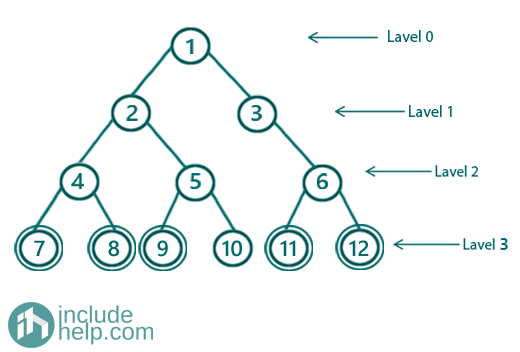# Sum of all nodes in a Binary Tree

In this article, we are going to see how to find the sum of all nodes in the given binary tree?
Submitted by Radib Kar, on August 21, 2020

Prerequisite: Pre-order traversal, Level order traversal

To find the sum of all nodes, we need to traverse the tree and keep adding each node while traversing.

Now, we can either traverse depth-first search wise (DFS type traversals like preorder, inorder, postorder) or breadth-first wise(Level order traversal)

Both will take O(n) if time complexity is considered and regarding space complexity, if we use breadth-first like traversal( level order) then we will use queue space. if we use depth-first based traversals( here we have used preorder, you can use whatever you want), then there is stack space for recursive calls.

For example,

The below tree has sum 78 for all nodesIn the below implementation, we show how we can sum using preorder traversal. To serve that purpose,

We use a variable named sum as a reference so that it will collect the node value and find the cumulative sum at each recursive call.

1) Depth first based traversal

```#include <bits/stdc++.h>
using namespace std;

// tree node is defined
class TreeNode {
public:
int val;
TreeNode* left;
TreeNode* right;
TreeNode(int data)
{
val = data;
left = NULL;
right = NULL;
}
};

//depth first traversal and summing
void dfs(TreeNode* root, int& sum)
{
if (!root)
return;

sum += root->val;
dfs(root->left, sum);
dfs(root->right, sum);
}

int sumofAllNodes(TreeNode* root)
{
int sum = 0;
dfs(root, sum);
return sum;
}
int main()
{
//building the tree like example
TreeNode* root = new TreeNode(1);
root->left = new TreeNode(2);
root->right = new TreeNode(3);
root->left->left = new TreeNode(4);
root->left->right = new TreeNode(5);
root->right->right = new TreeNode(6);
root->left->left->left = new TreeNode(7);
root->left->left->right = new TreeNode(8);
root->left->right->left = new TreeNode(9);
root->left->right->right = new TreeNode(10);
root->right->right->left = new TreeNode(11);
root->right->right->right = new TreeNode(12);

cout << "Sum of all nodes for the given tree is: " << sumofAllNodes(root) << endl;

return 0;
}
```

Output:

```Sum of all nodes for the given tree is: 78
```

Here, we use level order traversal, to sum up, all the nodes.

2) Breadth first based traversal

```#include <bits/stdc++.h>
using namespace std;

// tree node is defined
class TreeNode {
public:
int val;
TreeNode* left;
TreeNode* right;
TreeNode(int data)
{
val = data;
left = NULL;
right = NULL;
}
};

//breadth first traversal and summing
void bfs(TreeNode* root, int& sum)
{
if (!root)
return;

queue<TreeNode*> q;
q.push(root);
//do a level order traversal and keep adding node values
while (!q.empty()) {
TreeNode* temp = q.front();
q.pop();
//add current node value with sum
sum += temp->val;
if (temp->left) {
q.push(temp->left);
}
if (temp->right)
q.push(temp->right);
}
}

int sumofAllNodes(TreeNode* root)
{
int sum = 0;
bfs(root, sum);
return sum;
}

int main()
{
//building the tree like example
TreeNode* root = new TreeNode(1);
root->left = new TreeNode(2);
root->right = new TreeNode(3);
root->left->left = new TreeNode(4);
root->left->right = new TreeNode(5);
root->right->right = new TreeNode(6);
root->left->left->left = new TreeNode(7);
root->left->left->right = new TreeNode(8);
root->left->right->left = new TreeNode(9);
root->left->right->right = new TreeNode(10);
root->right->right->left = new TreeNode(11);
root->right->right->right = new TreeNode(12);

cout << "Sum of all nodes for the given tree is: " << sumofAllNodes(root) << endl;

return 0;
}
```

Output:

```Sum of all nodes for the given tree is: 78
```

What's New (MCQs)

Top Interview Coding Problems/Challenges!

Languages: » C » C++ » C++ STL » Java » Data Structure » C#.Net » Android » Kotlin » SQL
Web Technologies: » PHP » Python » JavaScript » CSS » Ajax » Node.js » Web programming/HTML
Solved programs: » C » C++ » DS » Java » C#
Aptitude que. & ans.: » C » C++ » Java » DBMS
Interview que. & ans.: » C » Embedded C » Java » SEO » HR
CS Subjects: » CS Basics » O.S. » Networks » DBMS » Embedded Systems » Cloud Computing
» Machine learning » CS Organizations » Linux » DOS
More: » Articles » Puzzles » News/Updates

© https://www.includehelp.com some rights reserved.